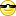FAQ/mse - CBU statistics Wiki

Revision 1 as of 2011-03-21 12:21:50

Clear message
location: FAQ / mse

# How do I compute Mean Square Error (MSE)?

Mean square error or MSE is frequently requested by journals as a companion statistic for ANOVA and, especially, t-tests. It is the average intra-group variance. MSE is outputted in ANOVA tables but can be computed using a weighted average of the group variances.

Fo G groups with the i-th an SD, $$SD_text{i}$$ and sample size $$N_text{i}$$,

MSE = $$\frac{\sum_text{i} (N_text{i}-1) SD^text{2}_{i}}{\sum_text{i} (N_text{i}-G) }$$

which equals $$\frac{\sum_text{i} SD^text{2}_{i}}{G}$$ in the sepcial case of equal group sizes.

In EXCEL, for two groups (A andin cells A1:A100 and B1:B100 respectively this corresponds to

MSE =(SQRT(((COUNT(A1:A100)-1)+(COUNT(B1:B100)-1)))*(AVERAGE(A1:A100)-AVERAGE(B1:B100)))/(SQRT((COUNT(A1:A100)-1)*POWER(STDEV(A1:A100),2)+(COUNT(B1:B100)-1)*POWER(STDEV(B1:B100),2)))